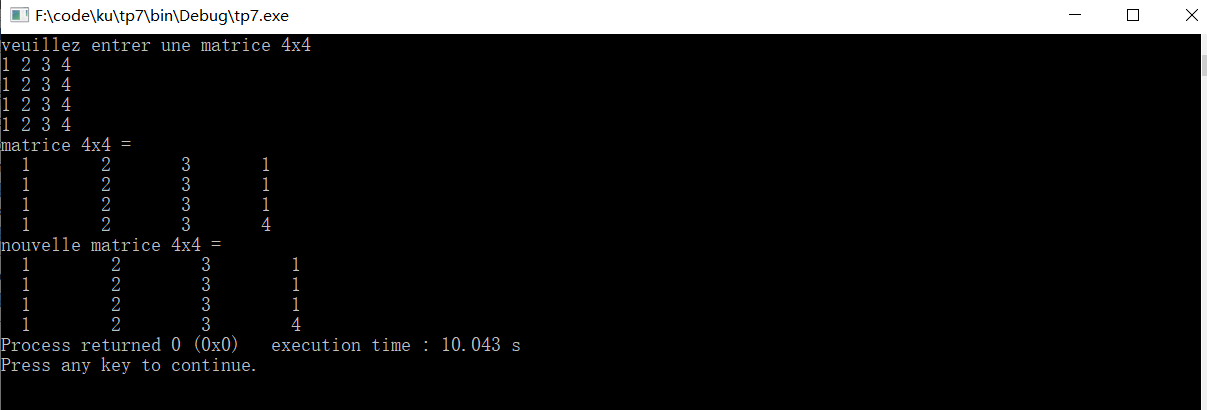# 一个3x3的数组无法正确获取数据？

``````#include <stdio.h>
#include <stdlib.h>
#include <time.h>
#define N 3
int affiche(int a[N][N])
{
for(int i=0;i<N+1;i++)
{
printf("\n");
for(int j=0;j<N+1;j++)
printf("%3d     ",a[i][j]);
}
}
int main()
{
int P[N];
int matrix;
printf("请输入一个 4x4 数组\n");
for(int i=0;i<4;i++)
{
for(int j=0;j<N+1;j++)
//matrix[i][j]=rand()%10;
scanf("%d",&matrix[i][j]);
}
printf("matrice 4x4 =");
affiche(matrix);``````

1 2 3 4

1 2 3 4

1 2 3 4

1 2 3 42020/11/27 19:40
• c语言
• 点赞
• 收藏
• 回答
私信

#### 1个回复